# Example Codes and Miniapps

This page provides a brief overview of MFEM's example codes and miniapps. For detailed documentation of the MFEM sources, including the examples, see the online Doxygen documentation, or the doc directory in the distribution.

The goal of the example codes is to provide a step-by-step introduction to MFEM in simple model settings. The miniapps are more complex, and are intended to be more representative of the advanced usage of the library in physics/application codes. We recommend that new users start with the example codes before moving to the miniapps.

Select from the categories below to display examples and miniapps that contain the respective feature. All examples support (arbitrarily) high-order meshes and finite element spaces. The numerical results from the example codes can be visualized using the GLVis visualization tool (based on MFEM). See the GLVis website for more details.

Users are encouraged to submit any example codes and miniapps that they have created and would like to share.
Contact a member of the MFEM team to report bugs or post questions or comments.

## Example 0: Simplest Laplace Problem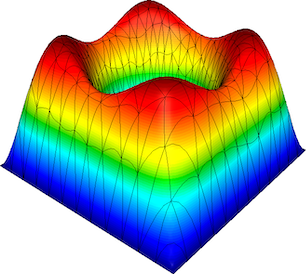This is the simplest MFEM example and a good starting point for new users. The example demonstrates the use of MFEM to define and solve an $H^1$ finite element discretization of the Laplace problem $$-\Delta u = 1 \quad\text{in } \Omega$$ with homogeneous Dirichlet boundary conditions $$u = 0 \quad\text{on } \partial\Omega$$

The example illustrates the use of the basic MFEM classes for defining the mesh, finite element space, as well as linear and bilinear forms corresponding to the left-hand side and right-hand side of the discrete linear system.

The example has serial (ex0.cpp) and parallel (ex0p.cpp) versions.

## Example 1: Laplace Problem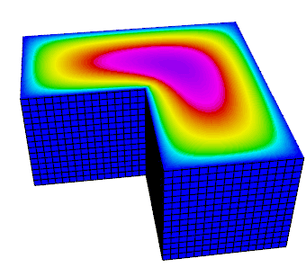This example code demonstrates the use of MFEM to define a simple isoparametric finite element discretization of the Laplace problem $$-\Delta u = 1$$ with homogeneous Dirichlet boundary conditions. Specifically, we discretize with the finite element space coming from the mesh (linear by default, quadratic for quadratic curvilinear mesh, NURBS for NURBS mesh, etc.) The problem solved in this example is the same as ex0, but with more sophisticated options and features.

The example highlights the use of mesh refinement, finite element grid functions, as well as linear and bilinear forms corresponding to the left-hand side and right-hand side of the discrete linear system. We also cover the explicit elimination of essential boundary conditions, static condensation, and the optional connection to the GLVis tool for visualization.

The example has a serial (ex1.cpp), a parallel (ex1p.cpp), and HPC versions: performance/ex1.cpp, performance/ex1p.cpp. It also has a PETSc modification in examples/petsc , a PUMI modification in examples/pumi and a Ginkgo modification in examples/ginkgo. Partial assembly and GPU devices are supported.

## Example 2: Linear Elasticity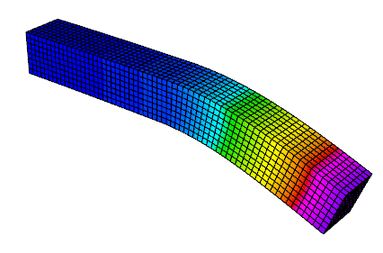This example code solves a simple linear elasticity problem describing a multi-material cantilever beam. Specifically, we approximate the weak form of $$-{\rm div}({\sigma}({\bf u})) = 0$$ where $${\sigma}({\bf u}) = \lambda\, {\rm div}({\bf u})\,I + \mu\,(\nabla{\bf u} + \nabla{\bf u}^T)$$ is the stress tensor corresponding to displacement field ${\bf u}$, and $\lambda$ and $\mu$ are the material Lame constants. The boundary conditions are ${\bf u}=0$ on the fixed part of the boundary with attribute 1, and ${\sigma}({\bf u})\cdot n = f$ on the remainder with $f$ being a constant pull down vector on boundary elements with attribute 2, and zero otherwise. The geometry of the domain is assumed to be as follows: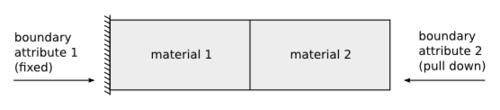The example demonstrates the use of high-order and NURBS vector finite element spaces with the linear elasticity bilinear form, meshes with curved elements, and the definition of piece-wise constant and vector coefficient objects. Static condensation is also illustrated.

The example has a serial (ex2.cpp) and a parallel (ex2p.cpp) version. It also has a PETSc modification in examples/petsc and a PUMI modification in examples/pumi. We recommend viewing Example 1 before viewing this example.

## Example 3: Definite Maxwell Problem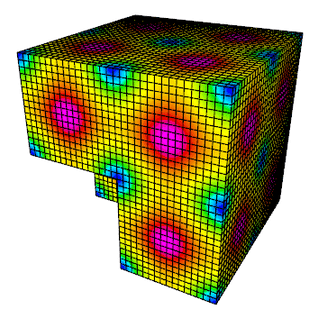This example code solves a simple 3D electromagnetic diffusion problem corresponding to the second order definite Maxwell equation $$\nabla\times\nabla\times\, E + E = f$$ with boundary condition $E \times n$ = "given tangential field". Here, we use a given exact solution $E$ and compute the corresponding r.h.s. $f$. We discretize with Nedelec finite elements in 2D or 3D.

The example demonstrates the use of $H(curl)$ finite element spaces with the curl-curl and the (vector finite element) mass bilinear form, as well as the computation of discretization error when the exact solution is known. Static condensation is also illustrated.

The example has a serial (ex3.cpp) and a parallel (ex3p.cpp) version. It also has a PETSc modification in examples/petsc. Partial assembly and GPU devices are supported. We recommend viewing examples 1-2 before viewing this example.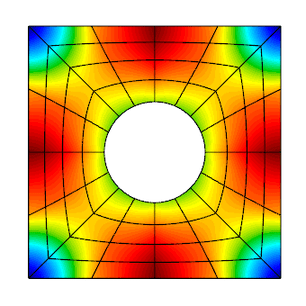This example code solves a simple 2D/3D $H(div)$ diffusion problem corresponding to the second order definite equation $$-{\rm grad}(\alpha\,{\rm div}(F)) + \beta F = f$$ with boundary condition $F \cdot n$ = "given normal field". Here we use a given exact solution $F$ and compute the corresponding right hand side $f$. We discretize with the Raviart-Thomas finite elements.

The example demonstrates the use of $H(div)$ finite element spaces with the grad-div and $H(div)$ vector finite element mass bilinear form, as well as the computation of discretization error when the exact solution is known. Bilinear form hybridization and static condensation are also illustrated.

The example has a serial (ex4.cpp) and a parallel (ex4p.cpp) version. It also has a PETSc modification in examples/petsc. Partial assembly and GPU devices are supported. We recommend viewing examples 1-3 before viewing this example.

## Example 5: Darcy Problem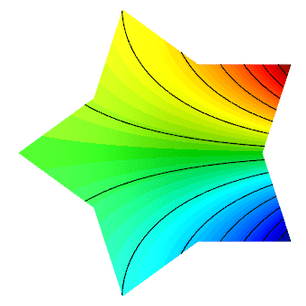This example code solves a simple 2D/3D mixed Darcy problem corresponding to the saddle point system $$\begin{array}{rcl} k\,{\bf u} + {\rm grad}\,p &=& f \\ -{\rm div}\,{\bf u} &=& g \end{array}$$ with natural boundary condition $-p =$ "given pressure". Here we use a given exact solution $({\bf u},p)$ and compute the corresponding right hand side $(f, g)$. We discretize with Raviart-Thomas finite elements (velocity $\bf u$) and piecewise discontinuous polynomials (pressure $p$).

The example demonstrates the use of the BlockMatrix and BlockOperator classes, as well as the collective saving of several grid functions in VisIt and ParaView formats.

The example has a serial (ex5.cpp) and a parallel (ex5p.cpp) version. It also has a PETSc modification in examples/petsc. Partial assembly is supported. We recommend viewing examples 1-4 before viewing this example.

## Example 6: Laplace Problem with AMR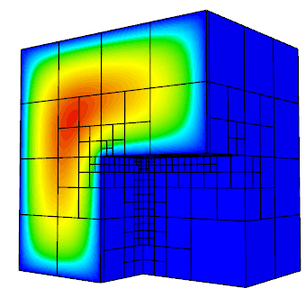This is a version of Example 1 with a simple adaptive mesh refinement loop. The problem being solved is again the Laplace equation $$-\Delta u = 1$$ with homogeneous Dirichlet boundary conditions. The problem is solved on a sequence of meshes which are locally refined in a conforming (triangles, tetrahedrons) or non-conforming (quadrilaterals, hexahedra) manner according to a simple ZZ error estimator.

The example demonstrates MFEM's capability to work with both conforming and nonconforming refinements, in 2D and 3D, on linear, curved and surface meshes. Interpolation of functions from coarse to fine meshes, as well as persistent GLVis visualization are also illustrated.

The example has a serial (ex6.cpp) and a parallel (ex6p.cpp) version. It also has a PETSc modification in examples/petsc and a PUMI modification in examples/pumi. Partial assembly and GPU devices are supported. We recommend viewing Example 1 before viewing this example.

## Example 7: Surface Meshes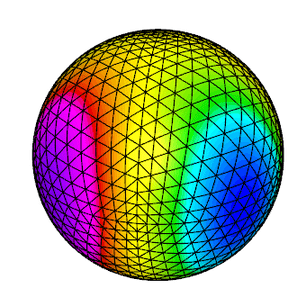This example code demonstrates the use of MFEM to define a triangulation of a unit sphere and a simple isoparametric finite element discretization of the Laplace problem with mass term, $$-\Delta u + u = f.$$

The example highlights mesh generation, the use of mesh refinement, high-order meshes and finite elements, as well as surface-based linear and bilinear forms corresponding to the left-hand side and right-hand side of the discrete linear system. Simple local mesh refinement is also demonstrated.

The example has a serial (ex7.cpp) and a parallel (ex7p.cpp) version. We recommend viewing Example 1 before viewing this example.

## Example 8: DPG for the Laplace Problem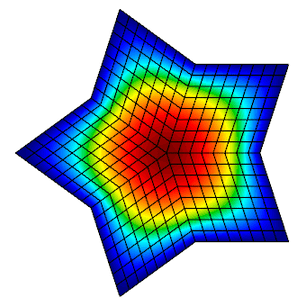This example code demonstrates the use of the Discontinuous Petrov-Galerkin (DPG) method in its primal 2x2 block form as a simple finite element discretization of the Laplace problem $$-\Delta u = f$$ with homogeneous Dirichlet boundary conditions. We use high-order continuous trial space, a high-order interfacial (trace) space, and a high-order discontinuous test space defining a local dual ($H^{-1}$) norm. We use the primal form of DPG, see "A primal DPG method without a first-order reformulation", Demkowicz and Gopalakrishnan, CAM 2013.

The example highlights the use of interfacial (trace) finite elements and spaces, trace face integrators and the definition of block operators and preconditioners.

The example has a serial (ex8.cpp) and a parallel (ex8p.cpp) version. We recommend viewing examples 1-5 before viewing this example.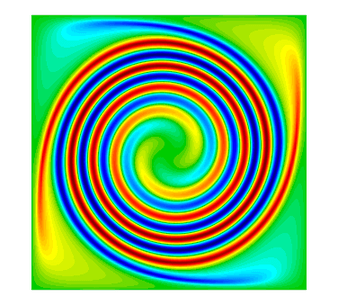This example code solves the time-dependent advection equation $$\frac{\partial u}{\partial t} + v \cdot \nabla u = 0,$$ where $v$ is a given fluid velocity, and $u_0(x)=u(0,x)$ is a given initial condition.

The example demonstrates the use of Discontinuous Galerkin (DG) bilinear forms in MFEM (face integrators), the use of explicit and implicit (with block ILU preconditioning) ODE time integrators, the definition of periodic boundary conditions through periodic meshes, as well as the use of GLVis for persistent visualization of a time-evolving solution. The saving of time-dependent data files for external visualization with VisIt and ParaView is also illustrated.

The example has a serial (ex9.cpp) and a parallel (ex9p.cpp) version. It also has a SUNDIALS modification in examples/sundials , a PETSc modification in examples/petsc, and a HiOp modification in examples/hiop.

## Example 10: Nonlinear Elasticity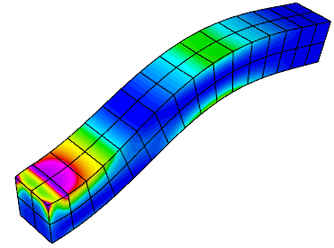This example solves a time dependent nonlinear elasticity problem of the form $$\frac{dv}{dt} = H(x) + S v\,,\qquad \frac{dx}{dt} = v\,,$$ where $H$ is a hyperelastic model and $S$ is a viscosity operator of Laplacian type. The geometry of the domain is assumed to be as follows: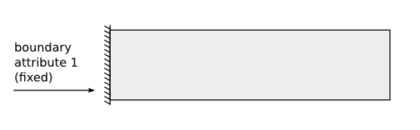The example demonstrates the use of nonlinear operators, as well as their implicit time integration using a Newton method for solving an associated reduced backward-Euler type nonlinear equation. Each Newton step requires the inversion of a Jacobian matrix, which is done through a (preconditioned) inner solver.

The example has a serial (ex10.cpp) and a parallel (ex10p.cpp) version. It also has a SUNDIALS modification in examples/sundials and a PETSc modification in examples/petsc. We recommend viewing examples 2 and 9 before viewing this example.

## Example 11: Laplace Eigenproblem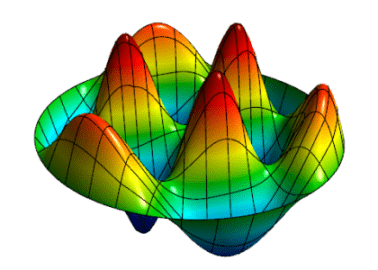This example code demonstrates the use of MFEM to solve the eigenvalue problem $$-\Delta u = \lambda u$$ with homogeneous Dirichlet boundary conditions.

We compute a number of the lowest eigenmodes by discretizing the Laplacian and Mass operators using a finite element space of the specified order, or an isoparametric/isogeometric space if order < 1 (quadratic for quadratic curvilinear mesh, NURBS for NURBS mesh, etc.)

The example highlights the use of the LOBPCG eigenvalue solver together with the BoomerAMG preconditioner in HYPRE, as well as optionally the SuperLU or STRUMPACK parallel direct solvers. Reusing a single GLVis visualization window for multiple eigenfunctions is also illustrated.

The example has only a parallel (ex11p.cpp) version. It also has a SLEPc modification in examples/petsc. We recommend viewing Example 1 before viewing this example.

## Example 12: Linear Elasticity Eigenproblem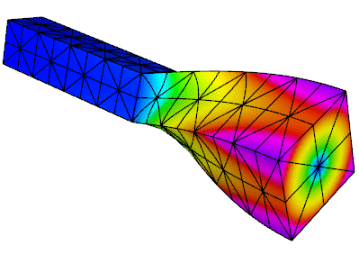This example code solves the linear elasticity eigenvalue problem for a multi-material cantilever beam. Specifically, we compute a number of the lowest eigenmodes by approximating the weak form of $$-{\rm div}({\sigma}({\bf u})) = \lambda {\bf u} \,,$$ where $${\sigma}({\bf u}) = \lambda\, {\rm div}({\bf u})\,I + \mu\,(\nabla{\bf u} + \nabla{\bf u}^T)$$ is the stress tensor corresponding to displacement field $\bf u$, and $\lambda$ and $\mu$ are the material Lame constants. The boundary conditions are ${\bf u}=0$ on the fixed part of the boundary with attribute 1, and ${\sigma}({\bf u})\cdot n = f$ on the remainder. The geometry of the domain is assumed to be as follows: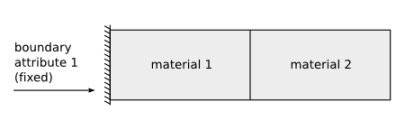The example highlights the use of the LOBPCG eigenvalue solver together with the BoomerAMG preconditioner in HYPRE. Reusing a single GLVis visualization window for multiple eigenfunctions is also illustrated.

The example has only a parallel (ex12p.cpp) version. We recommend viewing examples 2 and 11 before viewing this example.

## Example 13: Maxwell Eigenproblem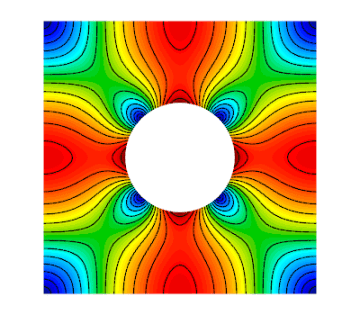This example code solves the Maxwell (electromagnetic) eigenvalue problem $$\nabla\times\nabla\times\, E = \lambda\, E$$ with homogeneous Dirichlet boundary conditions $E \times n = 0$.

We compute a number of the lowest nonzero eigenmodes by discretizing the curl curl operator using a Nedelec finite element space of the specified order in 2D or 3D.

The example highlights the use of the AME subspace eigenvalue solver from HYPRE, which uses LOBPCG and AMS internally. Reusing a single GLVis visualization window for multiple eigenfunctions is also illustrated.

The example has only a parallel (ex13p.cpp) version. We recommend viewing examples 3 and 11 before viewing this example.

## Example 14: DG Diffusion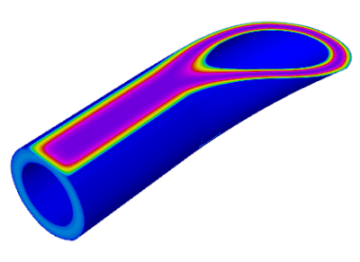This example code demonstrates the use of MFEM to define a discontinuous Galerkin (DG) finite element discretization of the Laplace problem $$-\Delta u = 1$$ with homogeneous Dirichlet boundary conditions. Finite element spaces of any order, including zero on regular grids, are supported. The example highlights the use of discontinuous spaces and DG-specific face integrators.

The example has a serial (ex14.cpp) and a parallel (ex14p.cpp) version. We recommend viewing examples 1 and 9 before viewing this example.

## Example 15: Dynamic AMR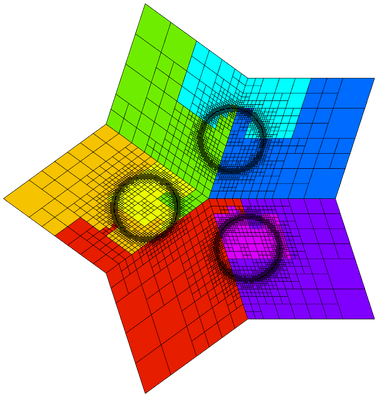Building on Example 6, this example demonstrates dynamic adaptive mesh refinement. The mesh is adapted to a time-dependent solution by refinement as well as by derefinement. For simplicity, the solution is prescribed and no time integration is done. However, the error estimation and refinement/derefinement decisions are realistic.

At each outer iteration the right hand side function is changed to mimic a time dependent problem. Within each inner iteration the problem is solved on a sequence of meshes which are locally refined according to a simple ZZ error estimator. At the end of the inner iteration the error estimates are also used to identify any elements which may be over-refined and a single derefinement step is performed. After each refinement or derefinement step a rebalance operation is performed to keep the mesh evenly distributed among the available processors.

The example demonstrates MFEM's capability to refine, derefine and load balance nonconforming meshes, in 2D and 3D, and on linear, curved and surface meshes. Interpolation of functions between coarse and fine meshes, persistent GLVis visualization, and saving of time-dependent fields for external visualization with VisIt are also illustrated.

The example has a serial (ex15.cpp) and a parallel (ex15p.cpp) version. We recommend viewing examples 1, 6 and 9 before viewing this example.

## Example 16: Time Dependent Heat Conduction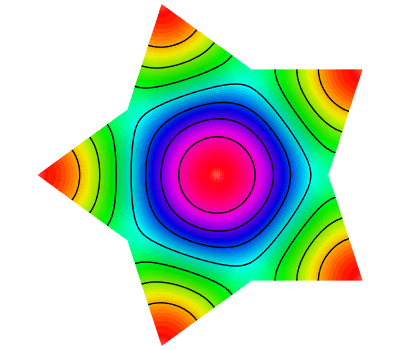This example code solves a simple 2D/3D time dependent nonlinear heat conduction problem $$\frac{du}{dt} = \nabla \cdot \left( \kappa + \alpha u \right) \nabla u$$ with a natural insulating boundary condition $\frac{du}{dn} = 0$. We linearize the problem by using the temperature field $u$ from the previous time step to compute the conductivity coefficient.

This example demonstrates both implicit and explicit time integration as well as a single Picard step method for linearization. The saving of time dependent data files for external visualization with VisIt is also illustrated.

The example has a serial (ex16.cpp) and a parallel (ex16p.cpp) version. We recommend viewing examples 2, 9, and 10 before viewing this example.

## Example 17: DG Linear Elasticity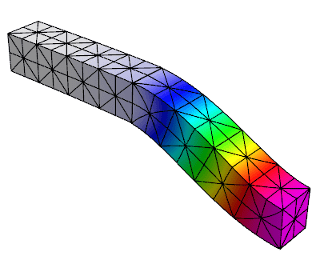This example code solves a simple linear elasticity problem describing a multi-material cantilever beam using symmetric or non-symmetric discontinuous Galerkin (DG) formulation.

Specifically, we approximate the weak form of $$-{\rm div}({\sigma}({\bf u})) = 0$$ where $${\sigma}({\bf u}) = \lambda\, {\rm div}({\bf u})\,I + \mu\,(\nabla{\bf u} + \nabla{\bf u}^T)$$ is the stress tensor corresponding to displacement field ${\bf u}$, and $\lambda$ and $\mu$ are the material Lame constants. The boundary conditions are Dirichlet, $\bf{u}=\bf{u_D}$, on the fixed part of the boundary, namely boundary attributes 1 and 2; on the rest of the boundary we use ${\sigma}({\bf u})\cdot n = {\bf 0}$. The geometry of the domain is assumed to be as follows: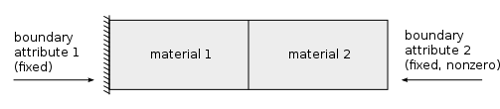The example demonstrates the use of high-order DG vector finite element spaces with the linear DG elasticity bilinear form, meshes with curved elements, and the definition of piece-wise constant and function vector-coefficient objects. The use of non-homogeneous Dirichlet b.c. imposed weakly, is also illustrated.

The example has a serial (ex17.cpp) and a parallel (ex17p.cpp) version. We recommend viewing examples 2 and 14 before viewing this example.

## Example 18: DG Euler Equations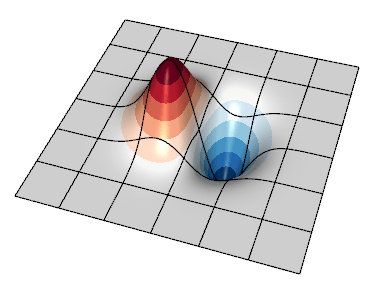This example code solves the compressible Euler system of equations, a model nonlinear hyperbolic PDE, with a discontinuous Galerkin (DG) formulation. The primary purpose is to show how a transient system of nonlinear equations can be formulated in MFEM. The equations are solved in conservative form

$$\frac{\partial u}{\partial t} + \nabla \cdot {\bf F}(u) = 0$$

with a state vector $u = [ \rho, \rho v_0, \rho v_1, \rho E ]$, where $\rho$ is the density, $v_i$ is the velocity in the $i^{\rm th}$ direction, $E$ is the total specific energy, and $H = E + p / \rho$ is the total specific enthalpy. The pressure, $p$ is computed through a simple equation of state (EOS) call. The conservative hydrodynamic flux ${\bf F}$ in each direction $i$ is

$${\bf F_{\it i}} = [ \rho v_i, \rho v_0 v_i + p \delta_{i,0}, \rho v_1 v_i + p \delta_{i,1}, \rho v_i H ]$$

Specifically, the example solves for an exact solution of the equations whereby a vortex is transported by a uniform flow. Since all boundaries are periodic here, the method's accuracy can be assessed by measuring the difference between the solution and the initial condition at a later time when the vortex returns to its initial location.

Note that as the order of the spatial discretization increases, the timestep must become smaller. This example currently uses a simple estimate derived by Cockburn and Shu for the 1D RKDG method. An additional factor can be tuned by passing the --cfl (or -c shorter) flag.

The example demonstrates user-defined bilinear and nonlinear form integrators for systems of equations that are defined with block vectors, and how these are used with an operator for explicit time integrators. In this case the system also involves an external approximate Riemann solver for the DG interface flux. It also demonstrates how to use GLVis for in-situ visualization of vector grid functions.

The example has a serial (ex18.cpp) and a parallel (ex18p.cpp) version. We recommend viewing examples 9, 14 and 17 before viewing this example.

## Example 19: Incompressible Nonlinear Elasticity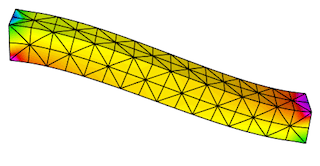This example code solves the quasi-static incompressible nonlinear hyperelasticity equations. Specifically, it solves the nonlinear equation $$\nabla \cdot \sigma(F) = 0$$ subject to the constraint $$\text{det } F = 1$$ where $\sigma$ is the Cauchy stress and $F_{ij} = \delta_{ij} + u_{i,j}$ is the deformation gradient. To handle the incompressibility constraint, pressure is included as an independent unknown $p$ and the stress response is modeled as an incompressible neo-Hookean hyperelastic solid. The geometry of the domain is assumed to be as follows: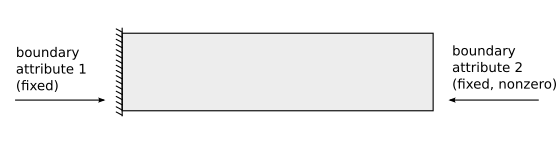This formulation requires solving the saddle point system $$\left[ \begin{array}{cc} K &B^T \\ B & 0 \end{array} \right] \left[\begin{array}{c} \Delta u \\ \Delta p \end{array} \right] = \left[\begin{array}{c} R_u \\ R_p \end{array} \right]$$ at each Newton step. To solve this linear system, we implement a specialized block preconditioner of the form $$P^{-1} = \left[\begin{array}{cc} I & -\tilde{K}^{-1}B^T \\ 0 & I \end{array} \right] \left[\begin{array}{cc} \tilde{K}^{-1} & 0 \\ 0 & -\gamma \tilde{S}^{-1} \end{array} \right]$$ where $\tilde{K}^{-1}$ is an approximation of the inverse of the stiffness matrix $K$ and $\tilde{S}^{-1}$ is an approximation of the inverse of the Schur complement $S = BK^{-1}B^T$. To approximate the Schur complement, we use the mass matrix for the pressure variable $p$.

The example demonstrates how to solve nonlinear systems of equations that are defined with block vectors as well as how to implement specialized block preconditioners for use in iterative solvers.

The example has a serial (ex19.cpp) and a parallel (ex19p.cpp) version. We recommend viewing examples 2, 5 and 10 before viewing this example.

## Example 20: Symplectic Integration of Hamiltonian SystemsThis example demonstrates the use of the variable order, symplectic time integration algorithm. Symplectic integration algorithms are designed to conserve energy when integrating systems of ODEs which are derived from Hamiltonian systems.

Hamiltonian systems define the energy of a system as a function of time (t), a set of generalized coordinates (q), and their corresponding generalized momenta (p). $$H(q,p,t) = T(p) + V(q,t)$$ Hamilton's equations then specify how q and p evolve in time: $$\frac{dq}{dt} = \frac{dH}{dp}\,,\qquad \frac{dp}{dt} = -\frac{dH}{dq}$$

To use the symplectic integration classes we need to define an mfem::Operator ${\bf P}$ which evaluates the action of dH/dp, and an mfem::TimeDependentOperator ${\bf F}$ which computes -dH/dq.

This example visualizes its results as an evolution in phase space by defining the axes to be $q$, $p$, and $t$ rather than $x$, $y$, and $z$. In this space we build a ribbon-like mesh with nodes at $(0,0,t)$ and $(q,p,t)$. Finally we plot the energy as a function of time as a scalar field on this ribbon-like mesh. This scheme highlights any variations in the energy of the system.

This example offers five simple 1D Hamiltonians:

• Simple Harmonic Oscillator (mass on a spring) $$H = \frac{1}{2}\left( \frac{p^2}{m} + \frac{q^2}{k} \right)$$
• Pendulum $$H = \frac{1}{2}\left[ \frac{p^2}{m} - k \left( 1 - cos(q) \right) \right]$$
• Gaussian Potential Well $$H = \frac{p^2}{2m} - k e^{-q^2 / 2}$$
• Quartic Potential $$H = \frac{1}{2}\left[ \frac{p^2}{m} + k \left( 1 + q^2 \right) q^2 \right]$$
• Negative Quartic Potential $$H = \frac{1}{2}\left[ \frac{p^2}{m} + k \left( 1 - \frac{q^2}{8} \right) q^2 \right]$$

In all cases these Hamiltonians are shifted by constant values so that the energy will remain positive. The mean and standard deviation of the computed energies at each time step are displayed upon completion.

When run in parallel, each processor integrates the same Hamiltonian system but starting from different initial conditions.

The example has a serial (ex20.cpp) and a parallel (ex20p.cpp) version. See the Maxwell miniapp for another application of symplectic integration.

## Example 21: Adaptive mesh refinement for linear elasticity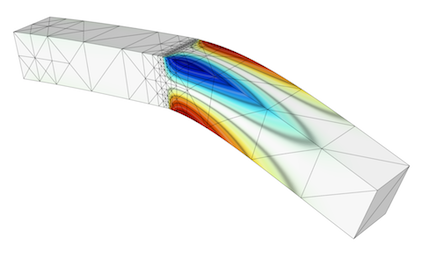This is a version of Example 2 with a simple adaptive mesh refinement loop. The problem being solved is again linear elasticity describing a multi-material cantilever beam. The problem is solved on a sequence of meshes which are locally refined in a conforming (triangles, tetrahedrons) or non-conforming (quadrilaterals, hexahedra) manner according to a simple ZZ error estimator.

The example demonstrates MFEM's capability to work with both conforming and nonconforming refinements, in 2D and 3D, on linear and curved meshes. Interpolation of functions from coarse to fine meshes, as well as persistent GLVis visualization are also illustrated.

The example has a serial (ex21.cpp) and a parallel (ex21p.cpp) version. We recommend viewing Examples 2 and 6 before viewing this example.

## Example 22: Complex Linear Systems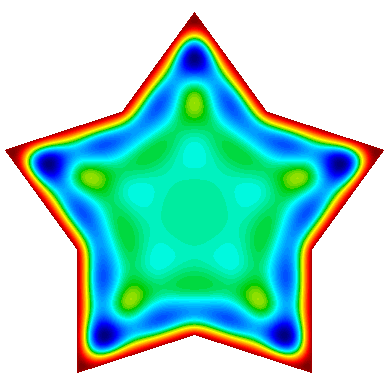This example code demonstrates the use of MFEM to define and solve a complex-valued linear system. It implements three variants of a damped harmonic oscillator:

• A scalar $H^1$ field: $$-\nabla\cdot\left(a \nabla u\right) - \omega^2 b\,u + i\,\omega\,c\,u = 0$$

• A vector $H(curl)$ field: $$\nabla\times\left(a\nabla\times\vec{u}\right) - \omega^2 b\,\vec{u} + i\,\omega\,c\,\vec{u} = 0$$

• A vector $H(div)$ field: $$-\nabla\left(a \nabla\cdot\vec{u}\right) - \omega^2 b\,\vec{u} + i\,\omega\,c\,\vec{u} = 0$$

In each case the field is driven by a forced oscillation, with angular frequency $\omega$, imposed at the boundary or a portion of the boundary.

The example also demonstrates how to display a time-varying solution as a sequence of fields sent to a single GLVis socket.

The example has a serial (ex22.cpp) and a parallel (ex22p.cpp) version. We recommend viewing examples 1, 3, and 4 before viewing this example.

## Example 23: Wave Problem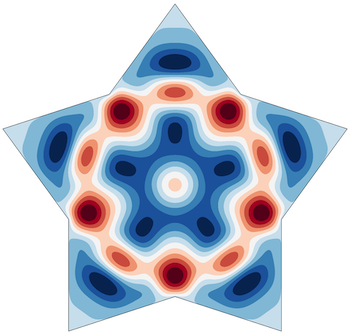This example code solves a simple 2D/3D wave equation with a second order time derivative: $$\frac{\partial^2 u}{\partial t^2} - c^2\Delta u = 0$$ The boundary conditions are either Dirichlet or Neumann.

The example demonstrates the use of time dependent operators, implicit solvers and second order time integration.

The example has only a serial (ex23.cpp) version. We recommend viewing examples 9 and 10 before viewing this example.

## Example 24: Mixed finite element spaces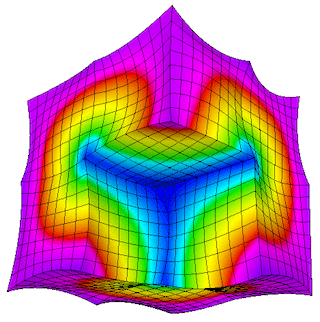This example code illustrates usage of mixed finite element spaces, with three variants:

• $H^1 \times H(curl)$
• $H(curl) \times H(div)$
• $H(div) \times L_2$

Using different approaches for demonstration purposes, we project or interpolate a gradient, curl, or divergence in the appropriate spaces, comparing the errors in each case.

Partial assembly and GPU devices are supported.

The example has a serial (ex24.cpp) and a parallel (ex24p.cpp) version. We recommend viewing examples 1 and 3 before viewing this example.

## Example 25: Perfectly Matched LayersThe example illustrates the use of a Perfectly Matched Layer (PML) for the simulation of time-harmonic electromagnetic waves propagating in unbounded domains.

PML was originally introduced by Berenger in "A Perfectly Matched Layer for the Absorption of Electromagnetic Waves". It is a technique used to solve wave propagation problems posed in infinite domains. The implementation involves the introduction of an artificial absorbing layer that minimizes undesired reflections. Inside this layer a complex coordinate stretching map is used which forces the wave modes to decay exponentially.

The example solves the indefinite Maxwell equations $$\nabla \times (a \nabla \times E) - \omega^2 b E = f.$$ where $a = \mu^{-1} |J|^{-1} J^T J$, $b= \epsilon |J| J^{-1} J^{-T}$ and $J$ is the Jacobian matrix of the coordinate transformation.

The example demonstrates discretization with Nedelec finite elements in 2D or 3D, as well as the use of complex-valued bilinear and linear forms. Several test problems are included, with known exact solutions.

The example has a serial (ex25.cpp) and a parallel (ex25p.cpp) version. We recommend viewing Example 22 before viewing this example.

## Example 26: Multigrid Preconditioner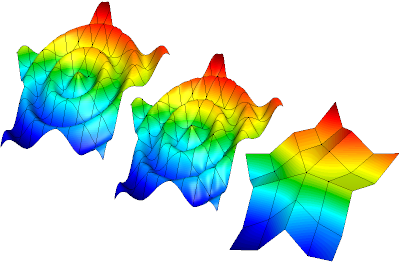This example code demonstrates the use of MFEM to define a simple isoparametric finite element discretization of the Laplace problem $$-\Delta u = 1$$ with homogeneous Dirichlet boundary conditions and how to solve it efficiently using a matrix-free multigrid preconditioner.

The example highlights on the creation of a hierarchy of discretization spaces and diffusion bilinear forms using partial assembly. The levels in the hierarchy of finite element spaces maybe constructed through geometric or order refinements. Moreover, the construction of a multigrid preconditioner for the PCG solver is shown. The multigrid uses a PCG solver on the coarsest level and second order Chebyshev accelerated smoothers on the other levels.

The example has a serial (ex26.cpp) and a parallel (ex26p.cpp) version. We recommend viewing Example 1 before viewing this example.

## Example 27: Laplace Boundary Conditions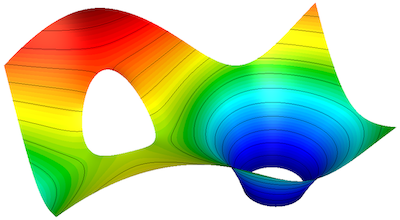This example code demonstrates the use of MFEM to define a simple finite element discretization of the Laplace problem: $$-\Delta u = 0$$ with a variety of boundary conditions.

Specifically, we discretize using a FE space of the specified order using a continuous or discontinuous space. We then apply Dirichlet, Neumann (both homogeneous and inhomogeneous), Robin, and Periodic boundary conditions on different portions of a predefined mesh.

Boundary conditions:
$u = u_{dbc}$ on $\Gamma_{dbc}$
$\hat{n}\cdot\nabla u = g_{nbc}$ on $\Gamma_{nbc}$
$\hat{n}\cdot\nabla u = 0$ on $\Gamma_{nbc_0}$
$\hat{n}\cdot\nabla u + a u = b$ on $\Gamma_{rbc}$

as well as periodic boundary conditions which are enforced topologically.

The example has a serial (ex27.cpp) and a parallel (ex27p.cpp) version. We recommend viewing examples 1 and 14 before viewing this example.

## Example 28: Constraints and Sliding Boundary Conditions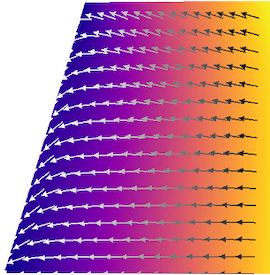This example code illustrates the use of constraints in linear solvers by solving an elasticity problem where the normal component of the displacement is constrained to zero on two boundaries but tangential displacement is allowed.

The constraints can be enforced in several different ways, including eliminating them from the linear system or solving a saddle-point system that explicitly includes constraint conditions.

The example has a serial (ex28.cpp) and a parallel (ex28p.cpp) version. We recommend viewing example 2 before viewing this example.

## Example 29: Solving PDEs on embedded surfaces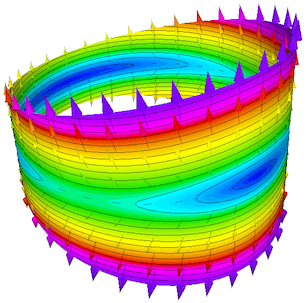This example demonstrates setting up and solving an anisotropic Laplace problem $$-\nabla\cdot(\sigma\nabla u) = 1 \quad\text{in } \Omega$$ with homogeneous Dirichlet boundary conditions $$u = 0 \quad\text{on } \partial\Omega$$ where $\Omega$ is a two dimensional curved surface embedded in three dimensions and $\sigma$ is an anisotropic diffusion tensor.

The example demonstrates and validates our DiffusionIntegrator's ability to properly integrate three dimensional fluxes on a two dimensional domain. Not all of our integrators currently support such cases but the DiffusionIntegrator can be used as a simple example of how extend other integrators when necessary.

The example has a serial (ex29.cpp) and a parallel (ex29p.cpp) version. We recommend viewing examples 1 and 7 before viewing this example.

## Example 30: Resolving rough and fine-scale problem data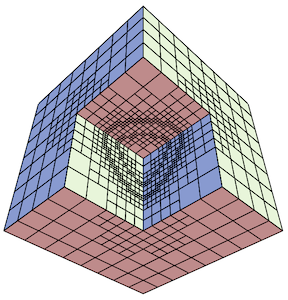Unresolved problem data will affect the accuracy of a discretized PDE solution as well as a posteriori estimates of the solution error. This example uses a CoefficientRefiner object to preprocess an input mesh until the resolution of the prescribed problem data $f \in L^2$ is below a prescribed tolerance. In this example, the resolution is identified with a data oscillation function on the mesh $\mathcal{T}$, defined $$\mathrm{osc}(f) = \Big( \sum_{T\in\mathcal{T}} \| h \cdot (I - \Pi)\, f \|^2_{L^2(T)} \Big)^{1/2},$$ where $h$ is the local element size function and $\Pi$ is a finite element projection operator, and the sum is taken over all elements $T$ in the mesh.

In this example, the coarse initial mesh is adaptively refined until $\mathrm{osc}(f)$ is below a prescribed tolerance for various candidate functions $f \in L^2$. When using rough problem data, it is recommended to perform this type of preprocessing before a posteriori error estimation.

The example has a serial (ex30.cpp) and a parallel (ex30p.cpp) version. We recommend viewing examples 1 and 6 before viewing this example.

## Example 31: Anisotropic Definite Maxwell Problem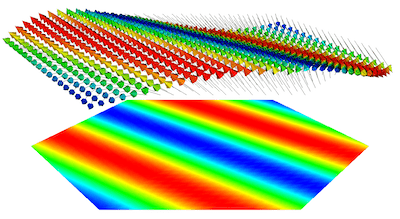This example code solves a simple electromagnetic diffusion problem corresponding to the second order definite Maxwell equation $$\nabla\times\nabla\times\, E + \sigma E = f$$ with boundary condition $E \times n$ = "given tangential field". In this example $\sigma$ is an anisotropic 3x3 tensor. Here, we use a given exact solution $E$ and compute the corresponding r.h.s. $f$. We discretize with Nedelec finite elements in 1D, 2D, or 3D.

The example demonstrates the use of restricted $H(curl)$ finite element spaces with the curl-curl and the (vector finite element) mass bilinear form, as well as the computation of discretization error when the exact solution is known. These restricted spaces allow the solution of 1D or 2D electromagnetic problems which involve 3D field vectors. Such problems arise in plasma physics and crystallography.

The example has a serial (ex31.cpp) and a parallel (ex31p.cpp) version. We recommend viewing example 3 before viewing this example.

## Example 32: Anisotropic Maxwell Eigenproblem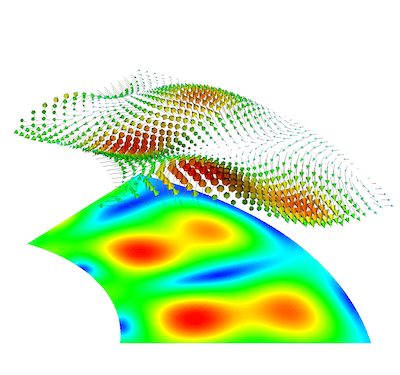This example code solves the Maxwell (electromagnetic) eigenvalue problem with anisotropic permittivity, $\epsilon$ $$\nabla\times\nabla\times\, E = \lambda\, \epsilon E$$ with homogeneous Dirichlet boundary conditions $E \times n = 0$.

We compute a number of the lowest nonzero eigenmodes by discretizing the curl curl operator using a Nedelec finite element space of the specified order in 1D, 2D, or 3D.

The example demonstrates the use of restricted $H(curl)$ finite element spaces in an eigenmode context. These restricted spaces allow the solution of 1D or 2D electromagnetic problems which involve 3D field vectors. Such problems arise in plasma physics and crystallography. The example highlights the use of the AME subspace eigenvalue solver from HYPRE, which uses LOBPCG and AMS internally. Reusing multiple GLVis visualization windows for multiple eigenfunctions is also illustrated.

The example has only a parallel (ex32p.cpp) version. We recommend viewing examples 13 and 31 before viewing this example.

## Example 33: Spectral fractional Laplacian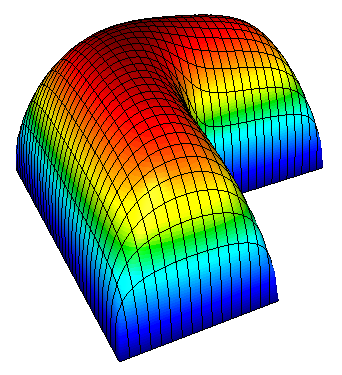This example code demonstrates the use of MFEM to solve the fractional Laplacian problem $$(-\Delta)^\alpha u = 1, \quad \alpha > 0,$$ with homogeneous Dirichlet boundary conditions. The problem solved in this example is similar to ex1, but involves a fractional-order diffusion operator whose inverse can be approximated by a series of inverses of integer-order diffusion operators. Solving each of these independent integer-order PDEs with MFEM and summing their solutions results in a discrete solution to the fractional Laplacian problem above.

The example has a serial (ex33.cpp) and a parallel (ex33p.cpp) version. We recommend viewing Example 1 before viewing this example.

## Volta Miniapp: Electrostatics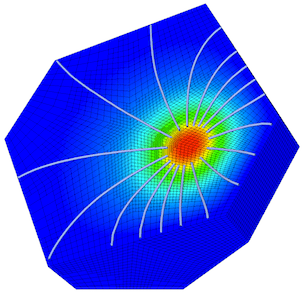This miniapp demonstrates the use of MFEM to solve realistic problems in the field of linear electrostatics. Its features include:

• dielectric materials
• charge densities
• surface charge densities
• prescribed voltages
• applied polarizations
• high order meshes
• high order basis functions

For more details, please see the documentation in the miniapps/electromagnetics directory.

The miniapp has only a parallel (volta.cpp) version. We recommend that new users start with the example codes before moving to the miniapps.

## Tesla Miniapp: Magnetostatics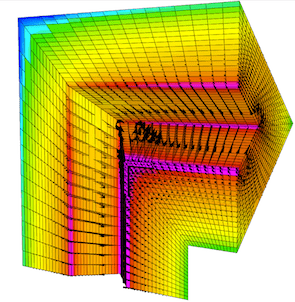This miniapp showcases many of MFEM's features while solving a variety of realistic magnetostatics problems. Its features include:

• diamagnetic and/or paramagnetic materials
• ferromagnetic materials
• volumetric current densities
• surface current densities
• external fields
• high order meshes
• high order basis functions

For more details, please see the documentation in the miniapps/electromagnetics directory.

The miniapp has only a parallel (tesla.cpp) version. We recommend that new users start with the example codes before moving to the miniapps.

## Maxwell Miniapp: Transient Full-Wave Electromagnetics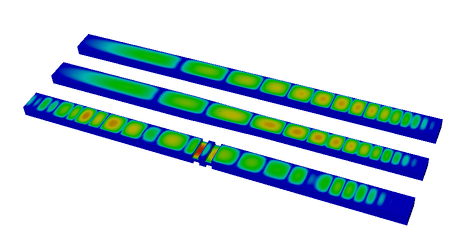This miniapp solves the equations of transient full-wave electromagnetics.

Its features include:

• mixed formulation of the coupled first-order Maxwell equations
• $H(\mathrm{curl})$ discretization of the electric field
• $H(\mathrm{div})$ discretization of the magnetic flux
• energy conserving, variable order, implicit time integration
• dielectric materials
• diamagnetic and/or paramagnetic materials
• conductive materials
• volumetric current densities
• Sommerfeld absorbing boundary conditions
• high order meshes
• high order basis functions

For more details, please see the documentation in the miniapps/electromagnetics directory.

The miniapp has only a parallel (maxwell.cpp) version. We recommend that new users start with the example codes before moving to the miniapps.

## Joule Miniapp: Transient Magnetics and Joule HeatingThis miniapp solves the equations of transient low-frequency (a.k.a. eddy current) electromagnetics, and simultaneously computes transient heat transfer with the heat source given by the electromagnetic Joule heating.

Its features include:

• $H^1$ discretization of the electrostatic potential
• $H(\mathrm{curl})$ discretization of the electric field
• $H(\mathrm{div})$ discretization of the magnetic field
• $H(\mathrm{div})$ discretization of the heat flux
• $L^2$ discretization of the temperature
• implicit transient time integration
• high order meshes
• high order basis functions

For more details, please see the documentation in the miniapps/electromagnetics directory.

The miniapp has only a parallel (joule.cpp) version. We recommend that new users start with the example codes before moving to the miniapps.

## Mobius Strip Miniapp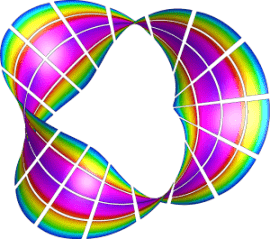This miniapp generates various Mobius strip-like surface meshes. It is a good way to generate complex surface meshes.

Manipulating the mesh topology and performing mesh transformation are demonstrated.

The mobius-strip mesh in the data directory was generated with this miniapp.

For more details, please see the documentation in the miniapps/meshing directory.

The miniapp has only a serial (mobius-strip.cpp) version. We recommend that new users start with the example codes before moving to the miniapps.

## Klein Bottle Miniapp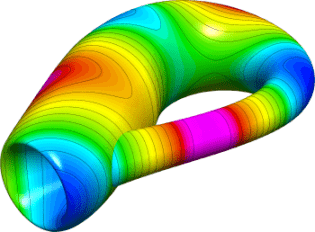This miniapp generates three types of Klein bottle surfaces. It is similar to the mobius-strip miniapp.

Manipulating the mesh topology and performing mesh transformation are demonstrated.

The klein-bottle and klein-donut meshes in the data directory were generated with this miniapp.

For more details, please see the documentation in the miniapps/meshing directory.

The miniapp has only a serial (klein-bottle.cpp) version. We recommend that new users start with the example codes before moving to the miniapps.

## Toroid Miniapp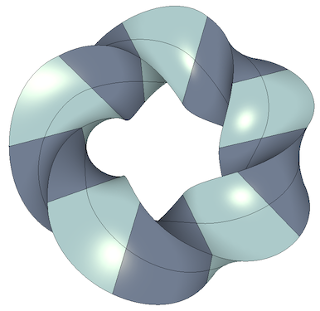This miniapp generates two types of toroidal volume meshes; one with triangular cross sections and one with square cross sections. It works by defining a stack of individual elements and bending them so that the bottom and top of the stack can be joined to form a torus. It supports various options including:

• The element type: 0 - Wedge, 1 - Hexahedron
• The geometric order of the elements
• The major and minor radii
• The number of elements in the azimuthal direction
• The number of nodes to offset by before rejoining the stack
• The initial angle of the cross sectional shape
• The number of uniform refinement steps to apply

Along with producing some visually interesting meshes, this miniapp demonstrates how simple 3D meshes can be constructed and transformed in MFEM. It also produces a family of meshes with simple but non-trivial topology for testing various features in MFEM.

This miniapp has only a serial (toroid.cpp) version. We recommend that new users start with the example codes before moving to the miniapps.

## Twist Miniapp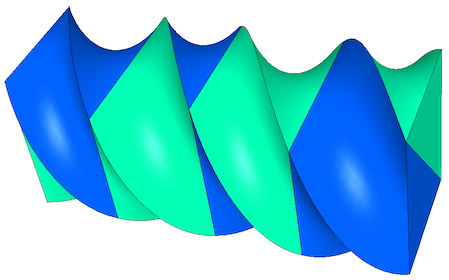This miniapp generates simple periodic meshes to demonstrate MFEM's handling of periodic domains. MFEM's strategy is to use a discontinuous vector field to define the mesh coordinates on a topologically periodic mesh. It works by defining a stack of individual elements and stitching together the top and bottom of the mesh. The stack can also be twisted so that the vertices of the bottom and top can be joined with any integer offset (for tetrahedral and wedge meshes only even offsets are supported).

The Twist miniapp supports various options including:

• The element type: 4 - Tetrahedron, 6 - Wedge, 8 - Hexahedron
• The geometric order of the elements
• The dimensions of the initial brick-shaped stack of elements
• The number of elements in the z direction
• The number of nodes to offset by before rejoining the stack
• The number of uniform refinement steps to apply

Along with producing some visually interesting meshes, this miniapp demonstrates how simple 3D meshes can be constructed and transformed in MFEM. It also produces a family of meshes with simple but non-trivial topology for testing various features in MFEM.

This miniapp has only a serial (twist.cpp) version. We recommend that new users start with the example codes before moving to the miniapps.

## Extruder Miniapp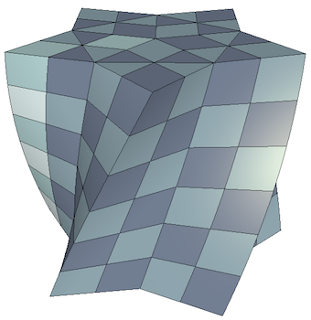This miniapp creates higher dimensional meshes from lower dimensional meshes by extrusion. Simple coordinate transformations can also be applied if desired.

• The initial mesh can be 1D or 2D
• 1D meshes can be extruded in both the y and z directions
• 2D meshes can be triangular, quadrilateral, or contain both element types
• Meshes with high order geometry are supported
• User can specify the number of elements and the distance to extrude
• Geometric order of the transformed mesh can be user selected or automatic

This miniapp provides another demonstration of how simple meshes can be constructed and transformed in MFEM.

This miniapp has only a serial (extruder.cpp) version. We recommend that new users start with the example codes before moving to the miniapps.

## Trimmer Miniapp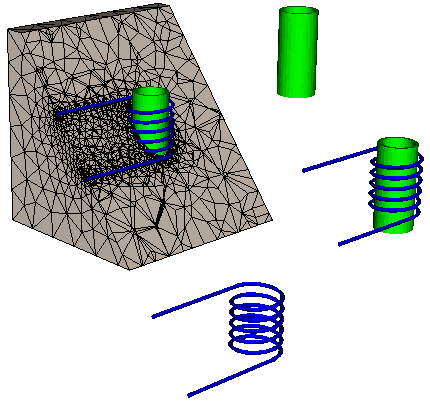This miniapp creates a new mesh file from an existing mesh by trimming away elements with selected attributes. Newly exposed boundary elements will be assigned new or user specified boundary attributes.

• The initial mesh can be 2D or 3D
• Meshes with high order geometry are supported
• Periodic meshes are supported
• NURBS meshes are not supported

This miniapp provides another demonstration of how simple meshes can be constructed in MFEM.

This miniapp has only a serial (trimmer.cpp) version. We recommend that new users start with the example codes before moving to the miniapps.

## Polar-NC Miniapp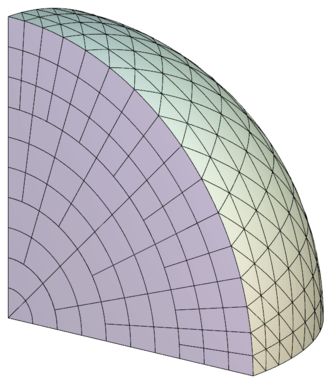This miniapp generates a circular sector mesh that consist of quadrilaterals and triangles of similar sizes. The 3D version of the mesh is made of prisms and tetrahedra.

The mesh is non-conforming by design, and can optionally be made curvilinear. The elements are ordered along a space-filling curve by default, which makes the mesh ready for parallel non-conforming AMR in MFEM.

The implementation also demonstrates how to initialize a non-conforming mesh on the fly by marking hanging nodes with Mesh::AddVertexParents.

For more details, please see the documentation in the miniapps/meshing directory.

The miniapp has only a serial (polar-nc.cpp) version. We recommend that new users start with the example codes before moving to the miniapps.

## Shaper Miniapp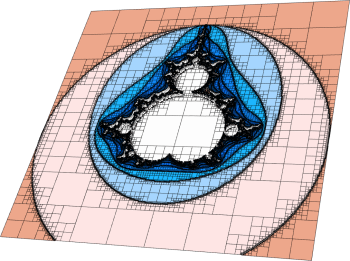This miniapp performs multiple levels of adaptive mesh refinement to resolve the interfaces between different "materials" in the mesh, as specified by a given material function.

It can be used as a simple initial mesh generator, for example in the case when the interface is too complex to describe without local refinement. Both conforming and non-conforming refinements are supported.

For more details, please see the documentation in the miniapps/meshing directory.

The miniapp has only a serial (shaper.cpp) version. We recommend that new users start with the example codes before moving to the miniapps.

## Mesh Explorer Miniapp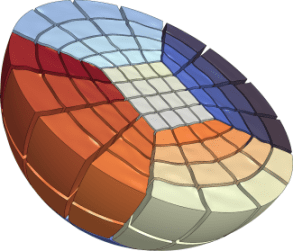This miniapp is a handy tool to examine, visualize and manipulate a given mesh. Some of its features are:

• visualizing of mesh materials and individual mesh elements
• mesh scaling, randomization, and general transformation
• manipulation of the mesh curvature
• the ability to simulate parallel partitioning
• quantitative and visual reports of mesh quality

For more details, please see the documentation in the miniapps/meshing directory.

The miniapp has only a serial (mesh-explorer.cpp) version. We recommend that new users start with the example codes before moving to the miniapps.

## Mesh Optimizer Miniapp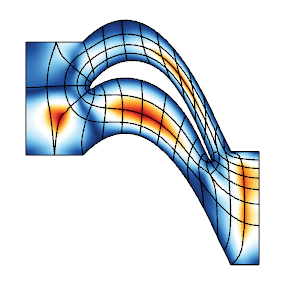This miniapp performs mesh optimization using the Target-Matrix Optimization Paradigm (TMOP) by P.Knupp et al., and a global variational minimization approach. It minimizes the quantity

$$\sum_T \int_T \mu(J(x)),$$

where $T$ are the target (ideal) elements, $J$ is the Jacobian of the transformation from the target to the physical element, and $\mu$ is the mesh quality metric.

This metric can measure shape, size or alignment of the region around each quadrature point. The combination of targets and quality metrics is used to optimize the physical node positions, i.e., they must be as close as possible to the shape / size / alignment of their targets.

This code also demonstrates a possible use of nonlinear operators, as well as their coupling to Newton methods for solving minimization problems. Note that the utilized Newton methods are oriented towards avoiding invalid meshes with negative Jacobian determinants. Each Newton step requires the inversion of a Jacobian matrix, which is done through an inner linear solver.

For more details, please see the documentation in the miniapps/meshing directory.

The miniapp has a serial (mesh-optimizer.cpp) and a parallel (pmesh-optimizer.cpp) version. We recommend that new users start with the example codes before moving to the miniapps.

## Minimal Surface Miniapp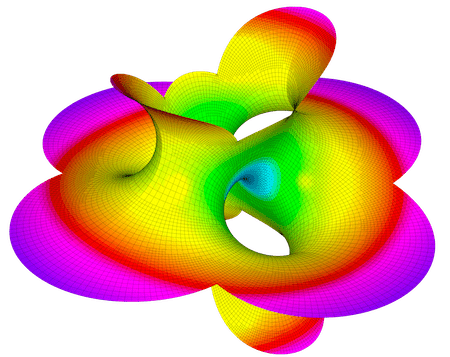This miniapp solves Plateau's problem: the Dirichlet problem for the minimal surface equation.

Options to solve the minimal surface equations of both parametric surfaces as well as surfaces restricted to be graphs of the form $z=f(x,y)$ are supported, including a number of examples such as the Catenoid, Helicoid, Costa and Schrek surfaces.

For more details, please see the documentation in the miniapps/meshing directory.

The miniapp has a serial (minimal-surface.cpp) and a parallel (pminimal-surface.cpp) version. We recommend that new users start with the example codes before moving to the miniapps.

## Low-Order Refined Transfer Miniapp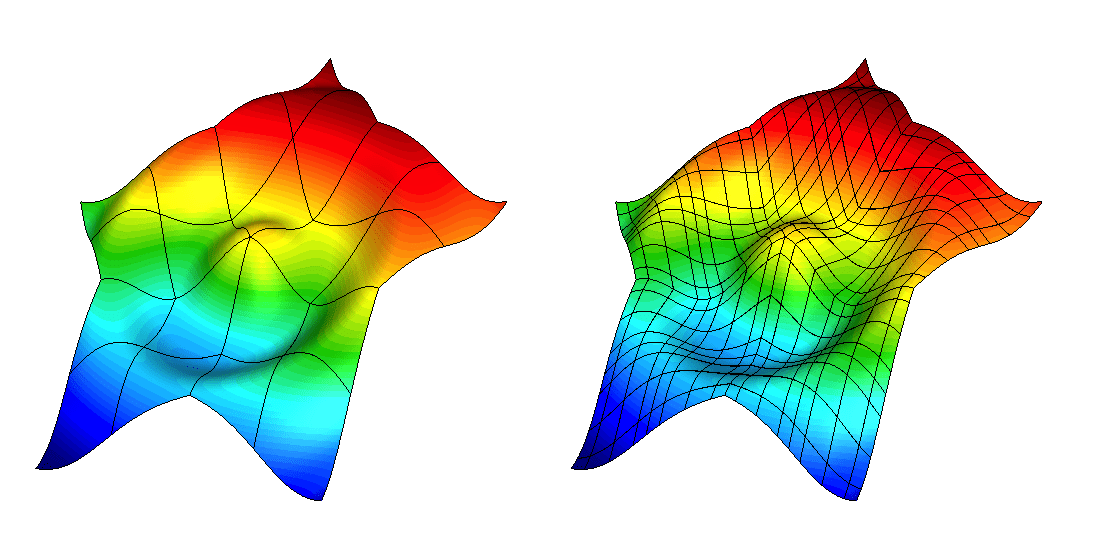The lor-transfer miniapp, found under miniapps/tools demonstrates the capability to generate a low-order refined mesh from a high-order mesh, and to transfer solutions between these meshes.

Grid functions can be transferred between the coarse, high-order mesh and the low-order refined mesh using either $L^2$ projection or pointwise evaluation. These transfer operators can be designed to discretely conserve mass and to recover the original high-order solution when transferring a low-order grid function that was obtained by restricting a high-order grid function to the low-order refined space.

The miniapp has only a serial (lor-transfer.cpp) version. We recommend that new users start with the example codes before moving to the miniapps.

## Interpolation Miniapps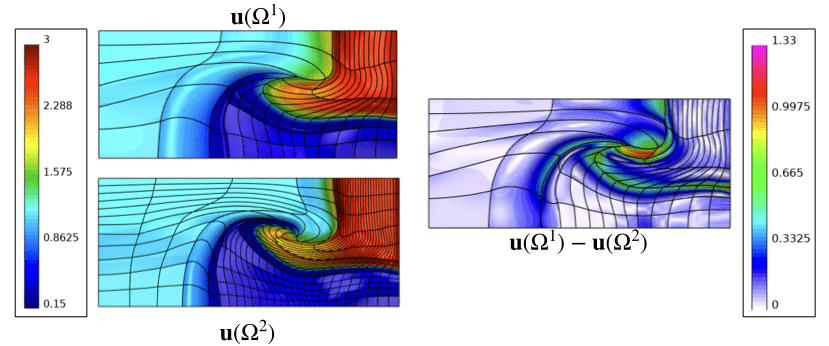The interpolation miniapp, found under miniapps/gslib, demonstrate the capability to interpolate high-order finite element functions at given set of points in physical space. These miniapps utilize the gslib library's high-order interpolation utility for quad and hex meshes:

• Find Points miniapp has a serial (findpts.cpp) and a parallel (pfindpts.cpp) version that demonstrate the basic procedures for point search and evaluation of grid functions.
• Field Interp miniapp (field-interp.cpp) demonstrates how grid functions can be transferred between meshes.
• Field Diff miniapp (field-diff.cpp) demonstrates how grid functions on two different meshes can be compared with each other.

These miniapps require installation of the gslib library. We recommend that new users start with the example codes before moving to the miniapps.

## Extrapolation Miniapp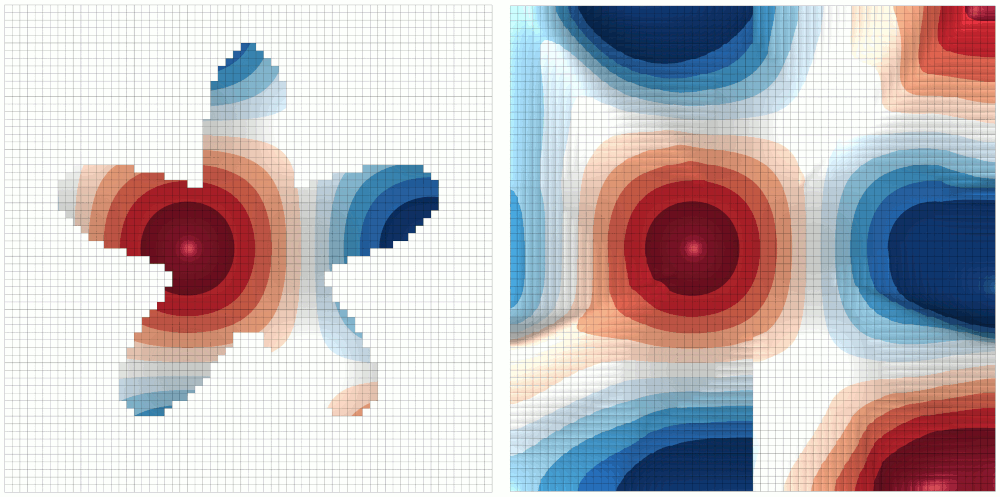The extrapolate miniapp, found in the miniapps/shifted directory, extrapolates a finite element function from a set of elements (known values) to the rest of the domain. The set of elements that contains the known values is specified by the positive values of a level set Coefficient. The known values are not modified. The miniapp supports two PDE-based approaches (Aslam, Bochkov & Gibou), both of which rely on solving a sequence of advection problems in the direction of the unknown parts of the domain. The extrapolation can be constant (1st order), linear (2nd order), or quadratic (3rd order). These formal orders hold for a limited band around the zero level set, see the above references for further information.

The miniapp has only a parallel (extrapolate.cpp) version. We recommend that new users start with the example codes before moving to the miniapps.

## Distance Solver Miniapp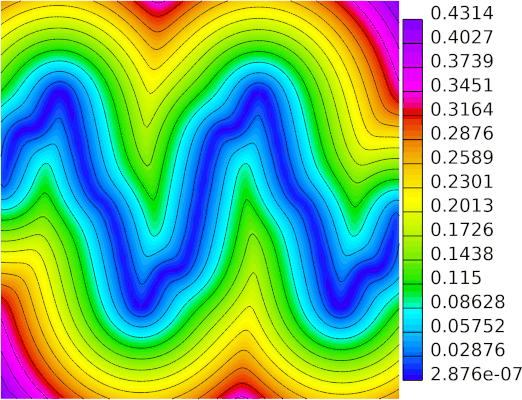The distance miniapp, found in the miniapps/shifted directory demonstrates the capability to compute the "distance" to a given point source or to the zero level set of a given function. Here "distance" refers to the length of the shortest path through the mesh. The input can be a DeltaCoefficient (representing a point source), or any Coefficient (for the case of a level set). The output is a ParGridFunction that can be scalar (representing the scalar distance), or a vector (its magnitude is the distance, and its direction is the starting direction of the shortest path).

The miniapp has only a parallel (distance.cpp) version. We recommend that new users start with the example codes before moving to the miniapps.

## Shifted Diffusion Miniapp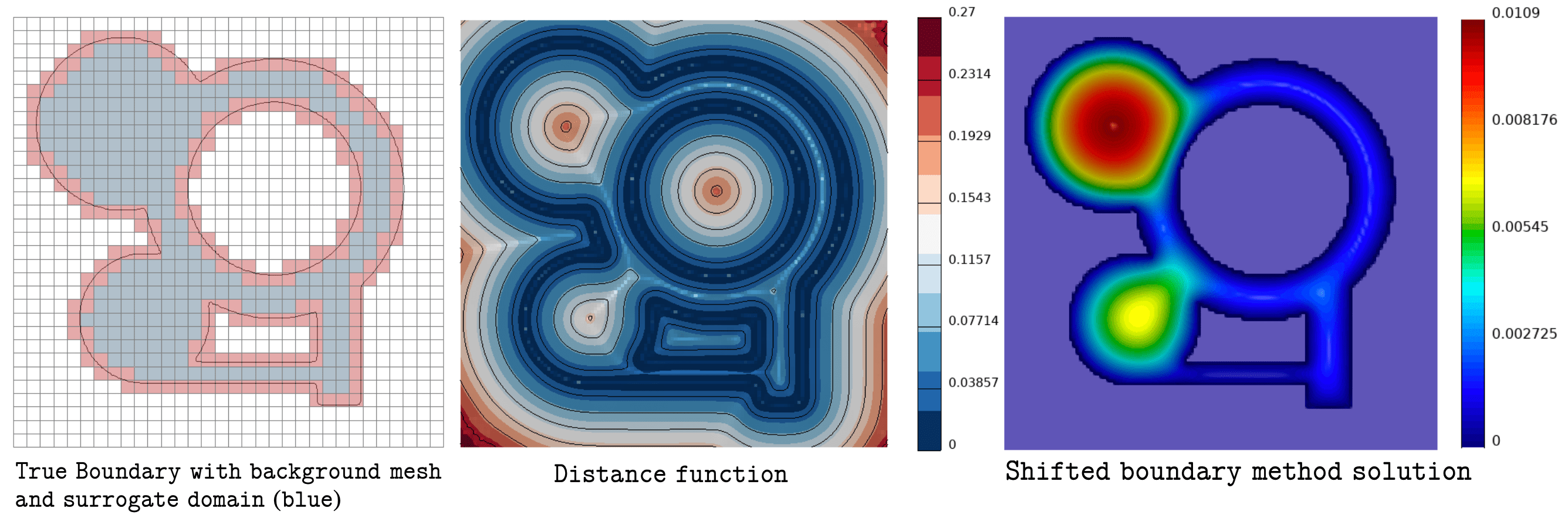The diffusion miniapp, found in the miniapps/shifted directory, demonstrates the capability to formulate a boundary value problem using a surrogate computational domain. The method uses a distance function to the true boundary to enforce Dirichlet boundary conditions on the (non-aligned) mesh faces, therefore "shifting" the location where boundary conditions are imposed. The implementation in the miniapp is a high-order extension of the second-generation shifted boundary method.

The miniapp has only a parallel (diffusion.cpp) version. We recommend that new users start with the example codes before moving to the miniapps.

## Laghos Miniapp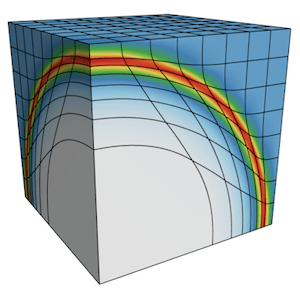Laghos (LAGrangian High-Order Solver) is a miniapp that solves the time-dependent Euler equations of compressible gas dynamics in a moving Lagrangian frame using unstructured high-order finite element spatial discretization and explicit high-order time-stepping.

The computational motives captured in Laghos include:

• Support for unstructured meshes, in 2D and 3D, with quadrilateral and hexahedral elements (triangular and tetrahedral elements can also be used, but with the less efficient full assembly option). Serial and parallel mesh refinement options can be set via a command-line flag.
• Explicit time-stepping loop with a variety of time integrator options. Laghos supports Runge-Kutta ODE solvers of orders 1, 2, 3, 4 and 6.
• Continuous and discontinuous high-order finite element discretization spaces of runtime-specified order.
• Moving (high-order) meshes.
• Separation between the assembly and the quadrature point-based computations.
• Point-wise definition of mesh size, time-step estimate and artificial viscosity coefficient.
• Constant-in-time velocity mass operator that is inverted iteratively on each time step. This is an example of an operator that is prepared once (fully or partially assembled), but is applied many times. The application cost is dominant for this operator.
• Time-dependent force matrix that is prepared every time step (fully or partially assembled) and is applied just twice per "assembly". Both the preparation and the application costs are important for this operator.
• Domain-decomposed MPI parallelism.
• Optional in-situ visualization with GLVis and data output for visualization / data analysis with VisIt.

The Laghos miniapp is part of the CEED software suite, a collection of software benchmarks, miniapps, libraries and APIs for efficient exascale discretizations based on high-order finite element and spectral element methods. See https://github.com/ceed for more information and source code availability.

This is an external miniapp, available at https://github.com/CEED/Laghos.

## Remhos MiniappRemhos (REMap High-Order Solver) is a miniapp that solves the pure advection equations that are used to perform monotonic and conservative discontinuous field interpolation (remap) as part of the Eulerian phase in Arbitrary Lagrangian Eulerian (ALE) simulations.

The computational motives captured in Remhos include:

• Support for unstructured meshes, in 2D and 3D, with quadrilateral and hexahedral elements. Serial and parallel mesh refinement options can be set via a command-line flag.
• Explicit time-stepping loop with a variety of time integrator options. Remhos supports Runge-Kutta ODE solvers of orders 1, 2, 3, 4 and 6.
• Discontinuous high-order finite element discretization spaces of runtime-specified order.
• Moving (high-order) meshes.
• Mass operator that is local per each zone. It is inverted by iterative or exact methods at each time step. This operator is constant in time (transport mode) or changing in time (remap mode). Options for full or partial assembly.
• Advection operator that couples neighboring zones. It is applied once at each time step. This operator is constant in time (transport mode) or changing in time (remap mode). Options for full or partial assembly.
• Domain-decomposed MPI parallelism.
• Optional in-situ visualization with GLVis and data output for visualization and data analysis with VisIt.

The Remhos miniapp is part of the CEED software suite, a collection of software benchmarks, miniapps, libraries and APIs for efficient exascale discretizations based on high-order finite element and spectral element methods. See https://github.com/ceed for more information and source code availability.

This is an external miniapp, available at https://github.com/CEED/Remhos.

## Block Solvers MiniappThe Block Solvers miniapp, found under miniapps/solvers, compares various linear solvers for the saddle point system obtained from mixed finite element discretization of the Darcy's flow problem \begin{array}{rcl} k{\bf u} & + \nabla p & = f \\ -\nabla \cdot {\bf u} & & = g \end{array}

The solvers being compared include:

• The divergence-free solver (couple and decoupled modes), which is based on a multilevel decomposition of the Raviart-Thomas finite element space and its divergence-free subspace.
• MINRES preconditioned by the block diagonal preconditioner in ex5p.cpp.

For more details, please see the documentation in the miniapps/solvers directory.

The miniapp supports:

• Arbitrary order mixed finite element pair (Raviart-Thomas elements + piecewise discontinuous polynomials)
• Various combination of essential and natural boundary conditions
• Homogeneous or heterogeneous scalar coefficient k

This miniapp has only a parallel (block-solvers.cpp) version. We recommend that new users start with the example codes before moving to the miniapps.

## Overlapping Grids Miniapps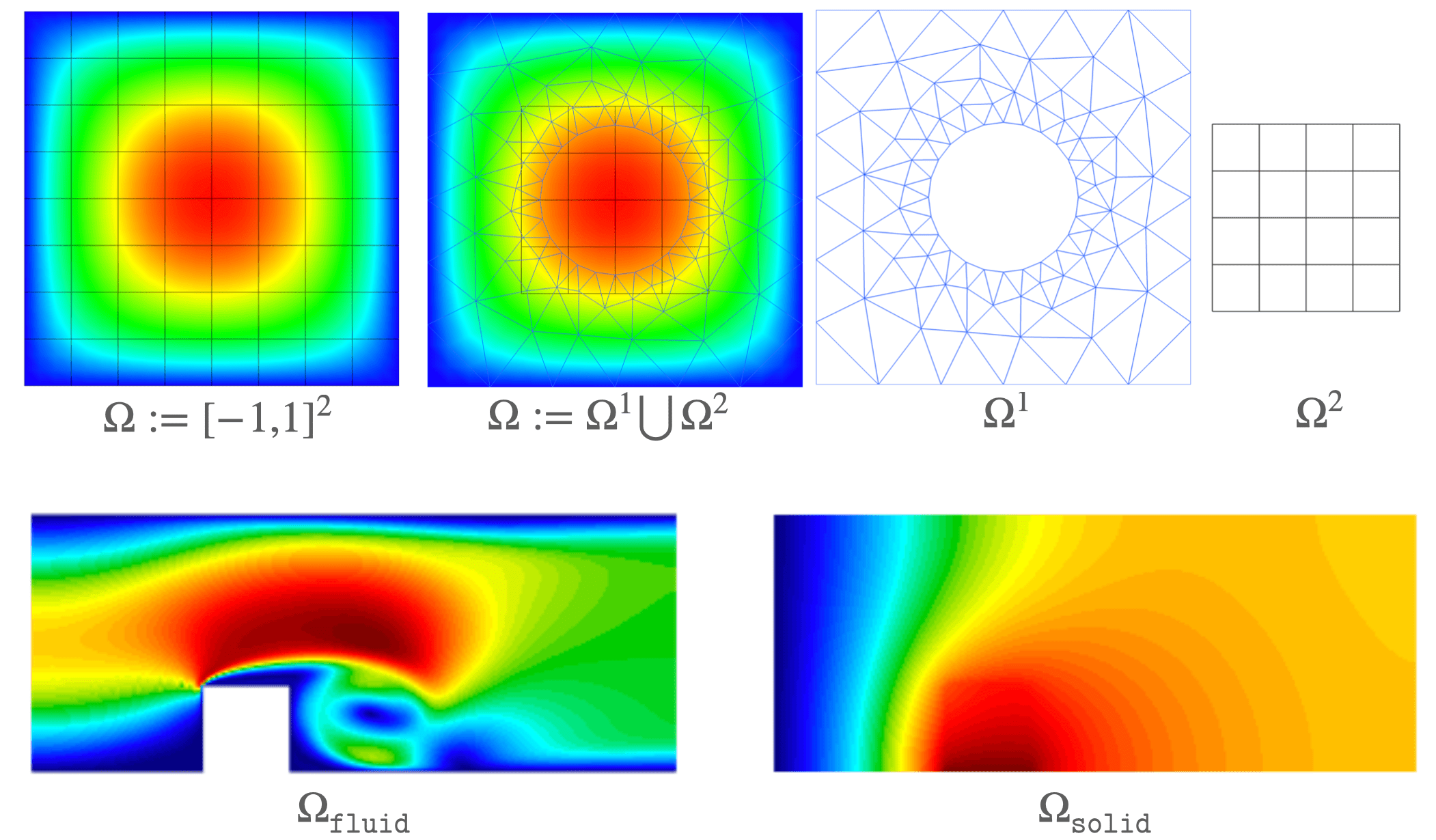Overlapping grids-based frameworks can often make problems tractable that are otherwise inaccessible with a single conforming grid. The following gslib-based miniapps in MFEM demonstrate how to set up and use overlapping grids:

• The Schwarz Example 1 miniapp in miniapps/gslib has a serial (schwarz_ex1.cpp) a parallel (schwarz_ex1p.cpp) version that solves the Poisson problem on overlapping grids. The serial version is restricted to use two overlapping grids, while the parallel version supports arbitrary number of overlapping grids.

• The Navier Conjugate Heat Transfer miniapp in miniapps/navier (navier_cht.cpp) demonstrates how a conjugate heat transfer problem can be solved with the fluid dynamics (incompressible Navier-Stokes equations) and heat transfer (advection-diffusion equation) PDEs modeled on different meshes.

These miniapps require installation of the gslib library. We recommend that new users start with the example codes before moving to the miniapps.

## ParELAG AMGe for H(curl) and H(div) MiniappThis is a miniapp that exhibits the ParELAG library and part of its capabilities. The miniapp employs MFEM and ParELAG to solve $H(\mathrm{curl})$- and $H(\mathrm{div})$-elliptic forms by an element based algebraic multigrid (AMGe).

ParELAG is a library mostly developed at the Center for Applied Scientific Computing of Lawrence Livermore National Laboratory, California, USA.

The miniapp uses:

• A multilevel hierarchy of de Rham complexes of finite element spaces, built by ParELAG;

• Hiptmair-type (hybrid) smoothers, implemented in ParELAG;

• AMS (Auxiliary-space Maxwell Solver) or ADS (Auxiliary-space Divergence Solver), from HYPRE, for preconditioning or solving on the coarsest levels.

Alternatively, it is possible to precondition or solve the $H(\mathrm{div})$ form on the coarsest level via a hybridization approach. However, this is not yet implemented in ParELAG for the coarse levels. Only the hybridization solver that is directly applicable to an $H(\mathrm{div})$-$L^2$ mixed (saddle-point) system is currently available in ParELAG.

We recommend viewing ex3p.cpp and ex4p.cpp before viewing this miniapp.

For more details, please see the documentation in the miniapps/parelag directory.

This miniapp has only a parallel (MultilevelHcurlHdivSolver.cpp) version. We recommend that new users start with the example codes before moving to the miniapps.

No examples or miniapps match your criteria.